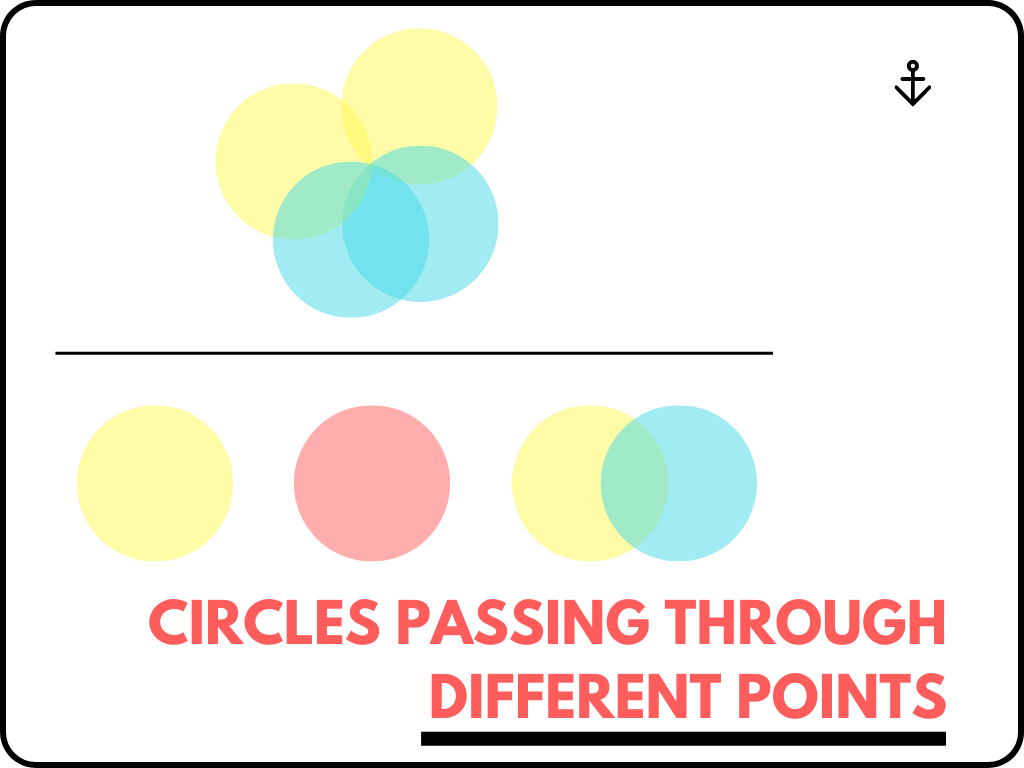## Circles Passing Through Different Points Class 10th

Circles Passing Through Different Points are as follows. Circles Passing Through One Point If there is a point in a plane then through that point many circles can pass. We can say infinite circles can pass. We can understand it practically from the figure given below. Circles Passing Through Two Points If there are two …